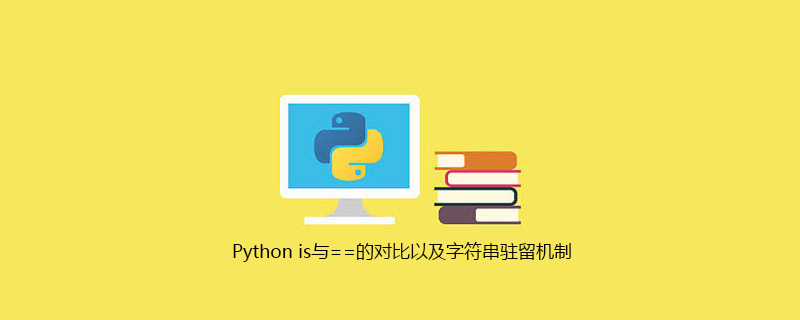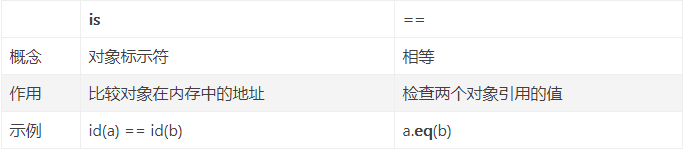# Python is与==的对比以及字符串驻留机制is 和 ==Python中的字符串采用了intern机制，当需要值相同的字符串的时候（比如标识符），可以直接从字符串池里拿来使用，避免频繁的创建和销毁，提升效率和节约内存，因此拼接和修改字符串是会比较影响性能的。因为是不可变的，所以字符串的操作都不是replace，而是新建对象，这也是为什么拼接多字符串的时候不建议用+而用join()，join()是先计算出所有字符串的长度，然后再拷贝，只new一次对象。需要注意的是，并不是所有的字符串都会采用intern机制，当且仅当只包含下划线、数字、字母的字符串才会被intern。

```a = "hello"
b = "hello"
print(a is b)  # 输出 True
print(a == b)  # 输出 True```

```a = "hello world"
b = "hello world"
print(a is b)  # 输出 False
print(a == b)  # 输出 True```

a和b中都有空格，所以不会被intern(空格不是python标识符)，故a和b不是同一个对象。注意，这仅仅是在交互式命令行中执行，而在PyCharm或者保存为文件执行，结果是不一样的，主要是因为解释器做了一部分优化。

```a = 'ab' + 'c' is 'abc'
print(a)  # 输出 True
ab = 'ab'
b = ab + 'c' is 'abc'
print(b)  # 输出 False```

```a = [1, 2, 3]
b = [1, 2, 3]
print(a is b)  # 输出 False
print(a == b)  # 输出 True```

a和b是列表，不是同一个对象。

```a = [1, 2, 3]
b = a
print(a is b)  # 输出 True
print(a == b)  # 输出 True```

```a = ["I", "love", "Python"]
b = a[:]
print(a is b)  # 输出 False
print(a == b)  # 输出 True
print(a is b)  # 输出 True
print(a == b)  # 输出 True```

b通过切片操作重新分配了对象(切片赋值)，但值和a相同。由于切片拷贝是浅拷贝，这说明列表中的元素并未重新创建，因此a is b输出为True。

```a = 1
b = 1
print(a is b)  # 输出 True
print(a == b)  # 输出 True```

Python会对比较小的整数对象进行缓存，下次用的时候直接从缓存中获取。

```a = 320
b = 320
print(a is b)  # 输出 False
print(a == b)  # 输出 True```

Python仅仅对比较小的整数对象进行缓存(范围为范围[-5, 256])，而并非是所有整数对象。注意，这仅仅是在交互式命令行中执行，而在PyCharm或者保存为文件执行，结果是不一样的，主要是因为解释器做了一部分优化。

is 与 == 对比

is 与 == 相比计算速度会更快，因为它不能重载，不用进行特殊的函数调用，通过直接比较两个整数 id，减少了函数调用的开销。而 a == b 则是等同于a.eq(b)，继承自 object 的 eq 方法原本也是比较两个对象的id，结果与 is 一样，但大多数Python对象会覆盖重写object的 eq 方法，而定义内容的相关比较，所以比较的是对象属性的值。

Python开发开发项目